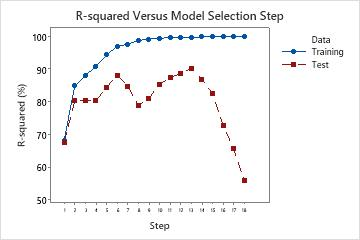# R-squared versus model selection step

When you use forward selection with validation as the stepwise procedure, Minitab provides a plot of the R2 statistic for the training data set and either the test R2 statistic or the k-fold stepwise R2 statistic for each step in the model selection procedure. The display of the test R2 statistic or the k-fold stepwise R2 statistic depends on whether you use a test data set or k-fold cross-validation.

## Interpretation

Use the plot to compare the values of the different R2 statistics at each step. Typically, the model performs well when the R2 statistics are both large. Minitab displays regression statistics for the model from the step that maximizes either the test R2 statistic or the k-fold stepwise R 2 statistic. The plot shows whether any simpler models fit well enough that they can also be good candidates.

In a case where the model is overfit, the test R2 statistic or the k-fold stepwise R2 statistic starts to decrease as terms enter the model. This decrease happens while the corresponding training R2 statistic or R2 statistic for all the data continues to increase. An over-fit model occurs when you add terms for effects that are not important in the population. An overfit model may not be useful for making predictions about the population. If a model is overfit, you can consider models from earlier steps.

The following plot shows test R2 as an example. Initially, the R2 statistics are both close to 70%. For the first few steps, the R2 statistics both tend to increase as terms enter the model. At step 6, the test R2 statistic is about 88%. The maximum value of the test R2 statistic is at step 14 and has a value close to 90%. You can consider whether the improvement in the fit justifies the additional complexity from adding more terms to the model.

After step 14, while the R2 continues to increase, the test R2 does not. The decrease in the test R2 after step 14 indicates that the model is overfit.By using this site you agree to the use of cookies for analytics and personalized content.  Read our policy